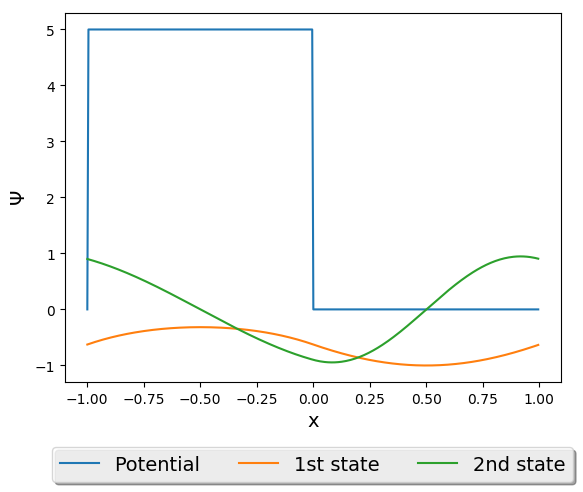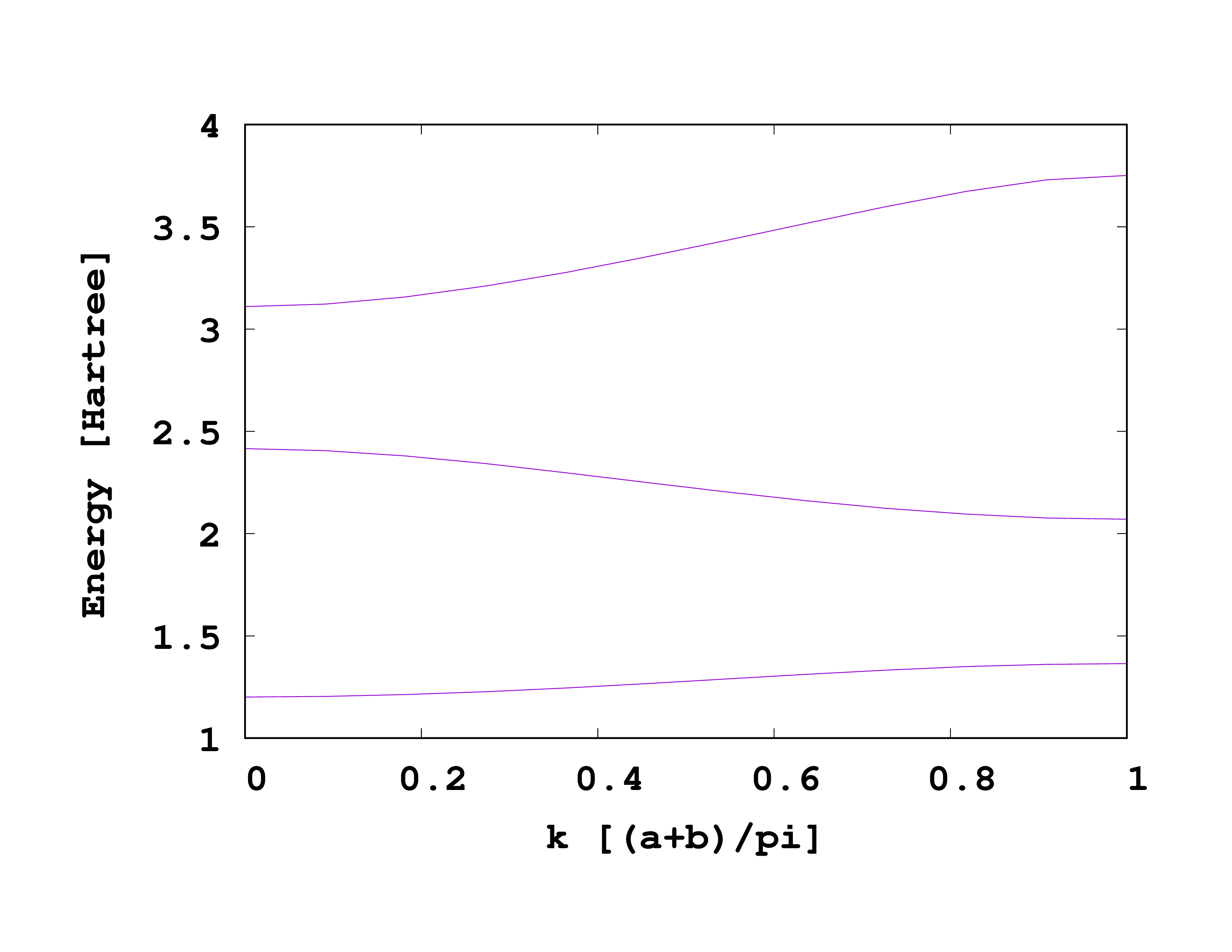# Kronig-Penney Model

The Kronig-Penney model is a 1D system that demonstrates band gaps, which relate to the allowed energies for electrons in a material. In this tutorial we calculate the bandstructure for Kronig-Penney Model. The Kronig-Penney Model has a periodic potential of

$$V(x) = \begin{cases} V_0 & -b < x < 0 \cr 0 & 0 < x < a \end{cases}$$

Where b is the width of each barrier, and a is the spacing between them.

## Input

The following input file will be used for the ground state calculation:



CalculationMode = gs
ExtraStates = 4
PeriodicDimensions = 1
Dimensions = 1
TheoryLevel = independent_particles

a = 5
b = 1
V = 3

Lsize = (a + b)/2

%LatticeParameters
a + b
%

Spacing = .0075

%Species
"A" | species_user_defined | potential_formula | "(x>-b)*V*(x<0)" | valence | 1
%

%Coordinates
"A" | 0 |
%

%KPointsGrid
11 |
%

%KPointsPath
11 |
0.0 |
0.5 |
%

ConvEigenError = trueThe first two wavefunctions plotted alongside the potential.

## Bandstructure

To calculate the bandstructure simply change the CalculationMode to unocc.



CalculationMode = unocc
ExtraStates = 4
PeriodicDimensions = 1
Dimensions = 1
TheoryLevel = independent_particles

a = 5
b = 1
V = 3

Lsize = (a + b)/2

%LatticeParameters
a + b
%

Spacing = .0075

%Species
"A" | species_user_defined | potential_formula | "(x>-b)*V*(x<0)" | valence | 1
%

%Coordinates
"A" | 0 |
%

%KPointsGrid
11 |
%

%KPointsPath
11 |
0.0 |
0.5 |
%

ConvEigenError = trueThe band structure for Kronig-Penney Model.

To plot the bandstructure, we will use the same command from the Periodic Systems (assuming you are using gnuplot).


plot for [col=5:5+9] 'static/bandstructure' u 1:(column(col)) w l notitle ls 1


Reference: Sidebottom DL. Fundamentals of condensed matter and crystalline physics: an introduction for students of physics and materials science. New York: Cambridge University Press; 2012.